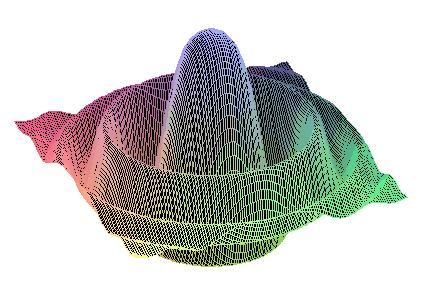DIANA Research Group
DIfferential Algebras and Nonlinear Analysis

Microlocal Analysis and Regularity Theory

 GF2009    Home    People    Research      Research Topics      Research Projects      START PROJECT      Related Research    Teaching      Current Teaching      Diploma Theses
 Regularity of solutions to partial differential equations (PDEs) is one of the central research issues in classical as well as modern analysis of partial differential operators (PDOs). By now, a far reaching regularity theory with powerful analytic methods is established in case of operators with (relatively) smooth coefficients. Furthermore, proofs of existence and uniqueness of solutions often rely substantially on such smoothness assumptions. One of the "key technologies" of modern regularity theory of PDEs is microlocal analysis. It combines information on the spatial concentration of singularities of a distributional solution with their spectral properties in the sense of Fourier analysis, leading to the notion of wave front set. In the context of bicharacteristics of PDOs microlocal analysis has become the crucial method in the study of propagation of singularities, which occur in a solution due to singularities in the given data (i.e., right-hand side or the initial values). Refined analytical techniques in determining microlocal regularity are based on the calculus of pseudodifferential operators and Fourier integral operators and their mapping properties with respect to wave front sets. Singularities of the given data for a PDE with smooth coefficients are typically reflected by a corresponding loss of regularity in the solution. This may limit the applicability of nonlinear operations, such as multiplications, involving the solution and distributional objects. In particular, this difficulty arises already when discussing solution concepts if we remove the smoothness constraints on the coefficients of the PDO. Situations of this kind appear naturally, for example, in models from the physics of wave propagation in complex media, although even in very simple cases the mere question of existence of solutions lies beyond the reach of distribution theory. One may then employ theories of algebras of generalized functions, in which such PDE problems become well-defined and are subject to a consistent extension of solvability theory. Specifically, with the type of partial (or pseudo) differential operators appearing in models of prominent applications, e.g., mathematical geophysics, a rigorous analysis has to concurrently allow for a wide range of singular coefficients (or symbols), highly singular initial values and source terms, generalized solutions, nonlinear differential-algebraic operations thereon. Within Colombeau algebras an intrinsic notion of regularity exists, which is compatible with the concept of smoothness of (embedded) distributions. By allowing for more general objects as solutions to PDEs, a natural further step is the extension of microlocal analysis to the context of generalized function algebras. In Colombeau theory this has been started in recent years, with successful case studies in applications to hyperbolic PDEs with discontinuous coefficients, theories of micro-hypoellipticity for linear PDOs with generalized function coefficients, propagation of singularities, and microlocal properties of basic nonlinear operations. On a refined level of quantitative regularity analysis, the influence of coefficient singularities on the solutions of PDEs is attempted to be measured in terms of generalized Hölder-Zygmund continuity. Here, the so-called Hölder-Zygmund classes of temperate distributions can be embedded into the broader nonlinear context of Colombeau algebras with an intrinsic, yet compatible, extension of their regularity scales. Primary new challenges in this whole analytical program are due to the interaction of the coefficient singularities with propagating singularities in the solution. A qualitatively new phenomenon to be taken into account here is the, classically unexpected, sensitivity of regularity properties to the lower order terms in the operators. A future comprehensive theory and the systematic development of refined tools in this direction has high potential for immediate applications in many models of mathematical physics (e.g. wave equations on singular curved space times, electrodynamics with interaction of singular charge distributions in complex media), and all problems involving wave propagation through highly irregular media (such as (non)linear elasticity, ocean acoustics, and seismology).
Last changed January 3, 2007 by M.K. and R.S.